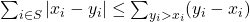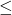# Tag Archives: Partition

## Randomization Test For Matched Pairs

This reduction gave me a lot of trouble and gave me a lot of holes to fill.  I think I filled them okay, but I’m getting the vibe that I’m missing something here- the reduction reads very differently from the way the problem is described.

The problem: Randomization Test For Matched Pairs.  This is problem MS10 in the appendix.

The description: Given a series of n pairs of integers (x1, y1) through (xn, yn), and a positive integer K.  Are there at least K subsets of the integers for which:?

Example:  The paper by Shamos that has the reduction might have a better explanation of what we are doing: he defines zi = yi – xi, and T* to be the sum of all of the positive zi (the right side of the inequality above).  Then if we could choose some set of zi to be positive (by changing their sign), and call that sum T, are there K or more sets where TT*?

So, suppose we have 4 pairs: (2,4), (1,6), (8,4), (3,2).  This would make our zi‘s {2,5,-4,-1}, and so T* would be 7.

There are 16 subsets of the above pairs (nothing seems to say that the empty set isn’t allowed).  Here are some whose T is7:

{(2,4), (1,6)}   (T=7)

{(2,4), (8,4)} (T=6)

{(2,4), (8,4), (3,2)} (T=7)

{(3,2)} (T=1)

..and so on.  A set that does not work is {(1,6), (8,4)} since its T is 9.

Reduction: Shamos uses Partition, so we are given a set of N elements.  The total sum of the elements is S, so we want two subsets of size S/2. He wants to “Perform the randomization test on the numbers” in the partition set, which I don’t get because the test needs to be done on pairs.  He also wants T* to be S/2.  The paper seems to say that you can just set that, but it has to be based on the zi.  So I came up with a way to make that happen:

For each item xi, create a pair  (xi, 0).  The zi for each of these elements will be negative.  Then add one extra pair (0. S/2).  Since this is the only positive z value, T* will be this value.  I don’t know if this is what the paper wants me to do, and I’m a little worried that adding an extra element will throw off what is next.

The paper then claims that if there is no partition of the set elements into equal-weight subsets, there are 2N-1 subsets with a T < T*.  This is because if there is no partition of equal size, then each subset of our elements either has a sum < S/2 (and this a T < T*), or its complement does.

If there is a partition, then 2 sets will have a T value of exactly T* (the two partition subsets) and half of the remaining subsets will have a T < T* as above.  So if we set K (the number of subsets whose T needs to be <= T*) to be 2N-1+2, we will have our reduction.

Difficulty: 7. I spent a long time trying to read and understand this reduction.  It’s very sparse, and, really, doesn’t explain at all how to make the pairs.  As a result, I’m pretty sure I filled in all of the holes, but it’s very possible that I’m missing something.

## Shapley-Shubik Voting Power

Setting up this definition will take a bit, bear with me.

The problem: Shapley-Shubik Voting Power.  This is problem MS8 in the appendix.

The description: Suppose we have a set of n voters, each voter i has a “weight” wi, corresponding to their number of votes.  If we are doing a simple majority vote, a coalition of voters then needs votes of (strictly) more than half the sum of the weights to win.  For a specific voter i, we can look at all permutations of voters, and say that all voters before voter i in the permutation voted “yes”, and all voters after voter i in the permutation voted “no”.  We are interested in the number of these permutations where voter i’s vote “matters”- where adding voter i to the “yes” votes makes “yes” the majority, but adding them to the “no” votes makes “no” the majority.  We call voter i a pivot player if this is the case.

Is voter 1 (in the G&J definition, it’s voter N in the paper) ever a pivot?

Some clarifications:  The number of times a voter is a pivot is the “Shapley-Shubik voting power”, and the proportion of permutations the voter is a pivot (by dividing the count by N!) is the “Shapley-Shubik power index”, but all we care about here is whether the power is non-zero.

Also, the definition of the voting game (in G&J, and also in the paper) allows for a more general definition of winning, besides a simple majority- you can supply a “quota” q, and any amount of votes ≥ q is a winning coalition.  For us, q is set in the reduction to be 1/2 the sum of the weights, +1, rounded up.

Example: Suppose we had 4 voters, with weights: 5.4,3,1.  There is 13 total weight, and 7 weight is enough to win. Voter 4 has 0 coalitions in which they are the pivot (we would need 6 votes without them), so has “zero Shapley-Shubik voting power”.

If, however, we split up the same number of votes among 5 voters: 4,3,3,2,1, then the 1-vote voter can be a pivot with (among others) the 4 and the 2.  The coalition loses without our pivot voter but wins with them.

Reduction: G&J have this as an “unpublished results” problem, but luckily I found a paper by Matsui and Matsui who solved it.  So we start with Partition.  We’re given a set A of n integers.  We will create a set of weights with one weight equal to each element of A, and add one extra voter with weight 1 at the end.  This is the voter that may or may not be a pivot.

If this last voter is a pivot, then there is a coalition that needs an additional weight of 1 to become a majority.  This means the coalition had exactly half of the votes without this voter (and so the sum of the elements is a partition of A)

In the other direction, if the set has a partition, then the partition set’s votes is equal to exactly half of the votes, one short of a majority.  Adding our 1-weight voter will make it a majority, and thus that voter is a pivot.

Difficulty: 4.  This problem looks scary because the definitions of Shapley-Shubik voting power include a lot more than what you actually need to do this reduction.  Once you get past all of that, it’s actually a pretty nice problem that students can handle.